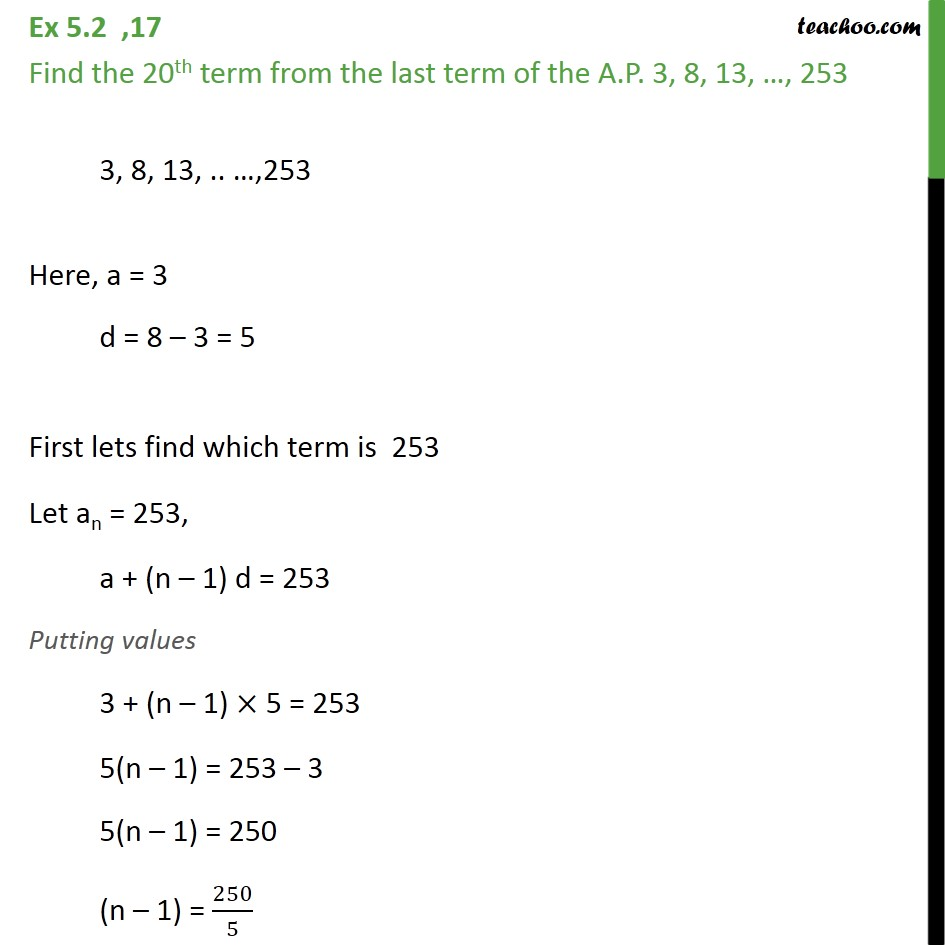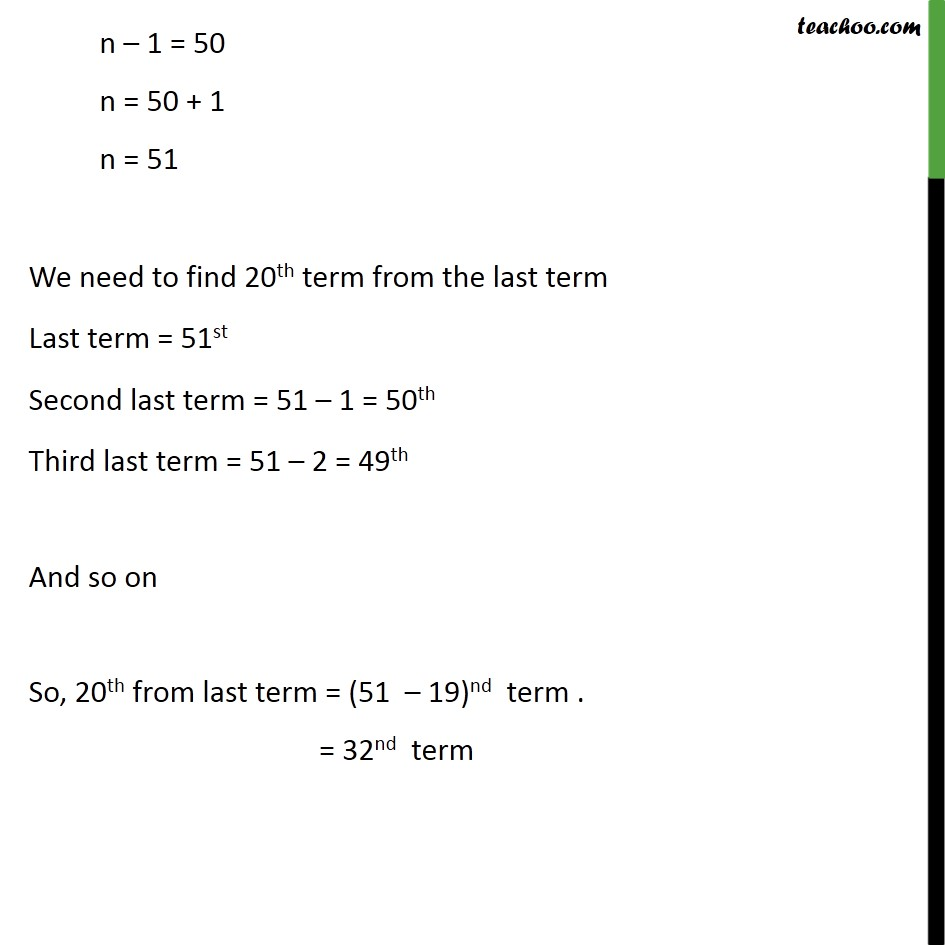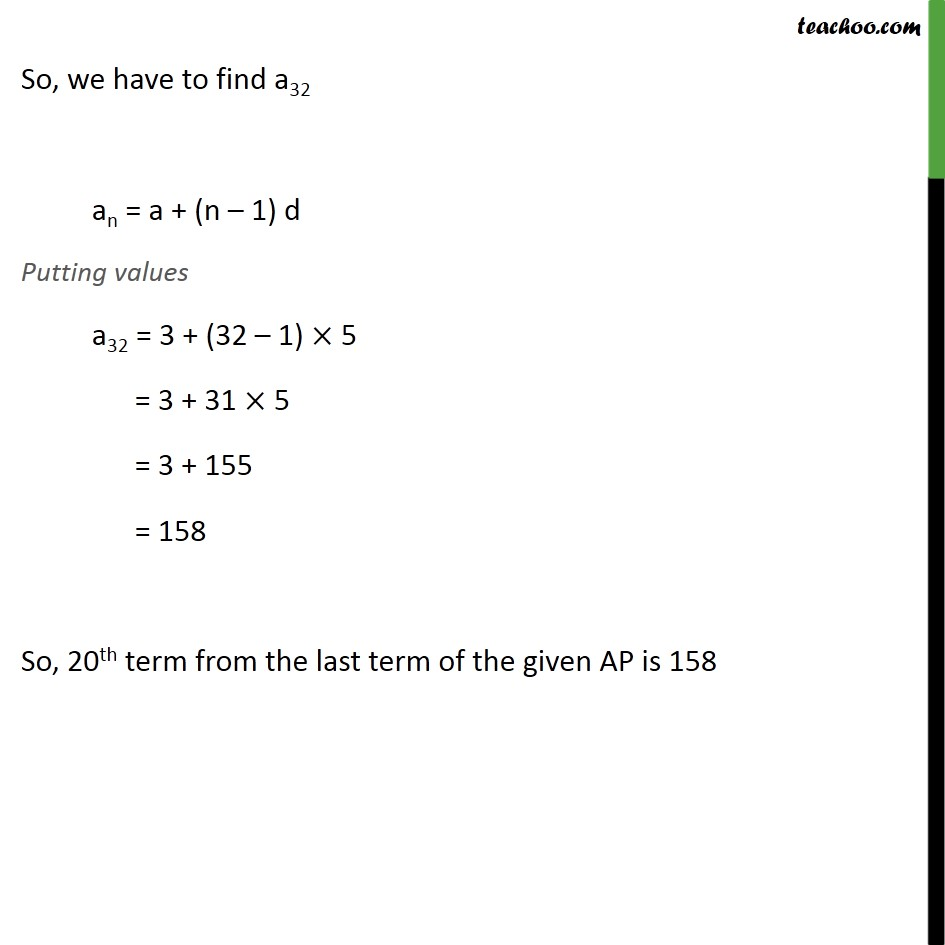Learn all Concepts of Chapter 5 Class 10 (with VIDEOS). Check - Arithmetic Progressions - Class 101. Chapter 5 Class 10 Arithmetic Progressions
2. Serial order wise
3. Ex 5.2

Transcript

Ex 5.2 ,17 Find the 20th term from the last term of the A.P. 3, 8, 13, , 253 3, 8, 13, .. ,253 Here, a = 3 d = 8 3 = 5 First lets find which term is 253 Let an = 253, a + (n 1) d = 253 Putting values 3 + (n 1) 5 = 253 5(n 1) = 253 3 5(n 1) = 250 (n 1) = 250/5 n 1 = 50 n = 50 + 1 n = 51 We need to find 20th term from the last term Last term = 51st Second last term = 51 1 = 50th Third last term = 51 2 = 49th And so on So, 20th from last term = (51 19)nd term . = 32nd term So, we have to find a32 an = a + (n 1) d Putting values a32 = 3 + (32 1) 5 = 3 + 31 5 = 3 + 155 = 158 So, 20th term from the last term of the given AP is 158

Ex 5.2